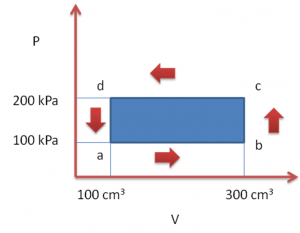# First law of Thermodynamics- FAQ in JEE

First law of thermodynamics is mainly based on the conservation of energy. Here we will look at what the law states and how it is applied.

## First Law Of Thermodynamics

Statement: The change in internal energy of a system is the heat added to the system minus the work done by the system.

Heat is a form of energy. There are two general ways of giving energy to a system:

1. By supplying heat to it.
2. By doing mechanical work on it.

### Example

Let us consider an ideal gas in a cylindrical container fitted with a piston. The piston is fixed to its position and the walls of the cylinder are at a higher temperature than the gas. Therefore the average kinetic energy of a wall molecule will be higher than the average kinetic energy of a gas molecule. So when the gas molecules strike the wall, they receive some energy from the wall molecules. Thus, the total internal energy of the gas increases.

Again, consider the same situation but now the walls are the same temperature as the gas. Suppose the piston is pushed inside very slowly to compress the gas. As the gas molecules collide with the piston, their speed increases. Hence the total internal energy of the gas increases.

Thus, the total internal energy of the gas increases when there is a heat transfer or when there is a work done on the gas.

If in a process, an amount ?Q of heat is given to the gas and an amount ?W of work is done by it. The total internal energy of the gas must then increase by ?Q – ?W.

?U = ?Q – ?W

This is the first law of thermodynamics.

### Conventions

When work is done by the system, ?W is positive and similarly when work is done on the system, ?W is negative. Also for heat given to the system, ?Q is positive and for heat given by the system, ?Q is negative.

Note: These conventions are valid only in Physics. In Chemistry we have different conventions.

### Work Done By A Gas

W = $int^{V_2}_{V_1} p dv$

Where

P is the pressure of the gas

V1 is the initial volume of the gas

V2 is the final volume of the gas

W = P (V2 –V1)

### Work Done Is An Isochoric Process

Since the volume remains constant, there is no work done by the system.

Thermodynamics Example: A thermodynamic system is taken through the cycle a-b-c-d-a as shown in the figure. Find the total heat rejected by the gas during the process.ab and cd are isobaric processes. So the work done can be calculated as:

For ab:

W = 100 (300 – 100) x 10-3 J = 20 J

For cd:

W = 200 (100 – 300) x 10-3 J = – 40 J/

bc and da are Isochoric processes. So the work done is zero.

Therefore total work done during the cycle:

?W = (20 – 40) J = – 20 J

The change in internal energy is zero as the initial state is same as the final state.

?U = 0

Using first law of thermodynamics

?U = ?Q – ?W

0 = ?Q – (- 20 J)

?Q = – 20 J

Therefore the system rejects 20 J of heat during the cycle.

To know more about the laws of thermodynamics, download BYJU’S learning app or visit or keep exploring the website for various information. Know All About JEE, JEE syllabus, JEE books, JEE Preparation tips etc.